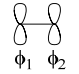# 1.7: Hückel Theory 2 (Eigenvalues)

$$\newcommand{\vecs}{\overset { \rightharpoonup} {\mathbf{#1}} }$$ $$\newcommand{\vecd}{\overset{-\!-\!\rightharpoonup}{\vphantom{a}\smash {#1}}}$$$$\newcommand{\id}{\mathrm{id}}$$ $$\newcommand{\Span}{\mathrm{span}}$$ $$\newcommand{\kernel}{\mathrm{null}\,}$$ $$\newcommand{\range}{\mathrm{range}\,}$$ $$\newcommand{\RealPart}{\mathrm{Re}}$$ $$\newcommand{\ImaginaryPart}{\mathrm{Im}}$$ $$\newcommand{\Argument}{\mathrm{Arg}}$$ $$\newcommand{\norm}{\| #1 \|}$$ $$\newcommand{\inner}{\langle #1, #2 \rangle}$$ $$\newcommand{\Span}{\mathrm{span}}$$ $$\newcommand{\id}{\mathrm{id}}$$ $$\newcommand{\Span}{\mathrm{span}}$$ $$\newcommand{\kernel}{\mathrm{null}\,}$$ $$\newcommand{\range}{\mathrm{range}\,}$$ $$\newcommand{\RealPart}{\mathrm{Re}}$$ $$\newcommand{\ImaginaryPart}{\mathrm{Im}}$$ $$\newcommand{\Argument}{\mathrm{Arg}}$$ $$\newcommand{\norm}{\| #1 \|}$$ $$\newcommand{\inner}{\langle #1, #2 \rangle}$$ $$\newcommand{\Span}{\mathrm{span}}$$$$\newcommand{\AA}{\unicode[.8,0]{x212B}}$$

The energies (eigenvalues) may be determined by using the Hückel approximation.$E \left( \psi_{B_{2g}} \right) = \dfrac{1}{6}(6)( \alpha - 2\beta ) = \alpha - 2\beta$

The energies of the remaining LCAO’s are:

$E \left( \psi_{E_{1g}}^a \right) = \left( \psi_{E_{1g}}^b \right) = \alpha + \beta$

$E \left( \psi_{E_{2u}}^a \right) = \left( \psi_{E_{2u}}^b \right) = \alpha - \beta$

Note the energies of the E orbitals are degenerate. Constructing the energy level diagram, we set α = 0 and β as the energy parameter (a negative quantity, so an MO whose energy is positive in units of β has an absolute energy that is negative),The energy of benzene based on the Hückel approximation is

$E_{total} = 2(2\beta) + 4(\beta) = 8\beta$

What is the delocalization energy (i.e. π resonance energy)?

To determine this, we consider cyclohexatriene, which is a six-membered cyclic ring with 3 localized π bonds; in other terms, cyclohexatriene is the product of three condensed ethylene molecules. For ethylene,Following the procedures outlined above, we find,\begin{aligned}
&\mathrm{E}\left(\psi_{1}\right)=\left\langle\frac{1}{\sqrt{2}}\left(\phi_{1}+\phi_{2}\right)|\mathrm{H}| \frac{1}{\sqrt{2}}\left(\phi_{1}+\phi_{2}\right)\right\rangle=\frac{1}{2}(2 \alpha+2 \beta)=\beta \\
&\mathrm{E}\left(\psi_{2}\right)=\left\langle\frac{1}{\sqrt{2}}\left(\phi_{1}-\phi_{2}\right)|\mathrm{H}| \frac{1}{\sqrt{2}}\left(\phi_{1}-\phi_{2}\right)\right\rangle=\frac{1}{2}(2 \alpha-2 \beta)=-\beta
\end{aligned}

The above was determined in the C2 point group. Correlating to D2h point group gives A in C2 → B1u in D2h and B in C2 → B2g in D2h:The Hückel energy of ethylene is,

$E_{total} = 2(\beta) = 2\beta$

Therefore, the energy of cyclohexatriene is 3(2β) = 6β. The resonance energy is therefore,The bond order is given by,Consider the B.O. between the C1 and C2 carbons of benzene

$[ \psi_{1}(A_{2u})] = 2( \dfrac{1}{ \sqrt{6}} )( \dfrac{1}{ \sqrt{6}}) = \dfrac{1}{3}$

$[ \psi_{3}(E_{1g}^a)] = 2( \dfrac{1}{ \sqrt{12}} )( \dfrac{1}{ \sqrt{12}}) = \dfrac{1}{3}$

$[ \psi_{4}(E_{1g}^b)] = \dfrac{1}{2}(0)( \dfrac{1}{2} ) = \dfrac{0}{ \dfrac{2}{3} }$This page titled 1.7: Hückel Theory 2 (Eigenvalues) is shared under a CC BY-NC-SA 4.0 license and was authored, remixed, and/or curated by Daniel Nocera via source content that was edited to the style and standards of the LibreTexts platform; a detailed edit history is available upon request.